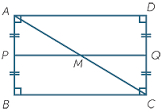# Rectangle 49153

Rectangle ABCD, whose | AB | = 5cm, | AC | = 8 cm, ∢ | CAB | = 30 °. How long is the other party and what is its area?

b =  4 cm
S =  20 cm2

### Step-by-step explanation:Did you find an error or inaccuracy? Feel free to write us. Thank you!

Tips to related online calculators
Pythagorean theorem is the base for the right triangle calculator.
Most natural application of trigonometry and trigonometric functions is a calculation of the triangles. Common and less common calculations of different types of triangles offers our triangle calculator. Word trigonometry comes from Greek and literally means triangle calculation.

#### You need to know the following knowledge to solve this word math problem:

We encourage you to watch this tutorial video on this math problem: